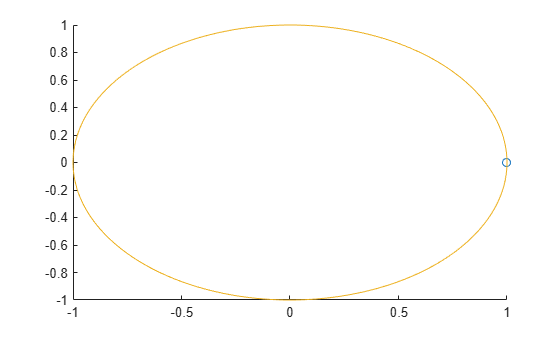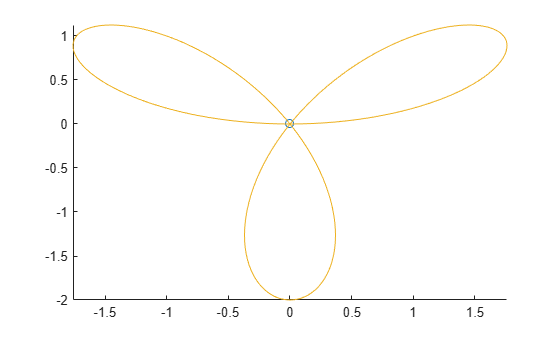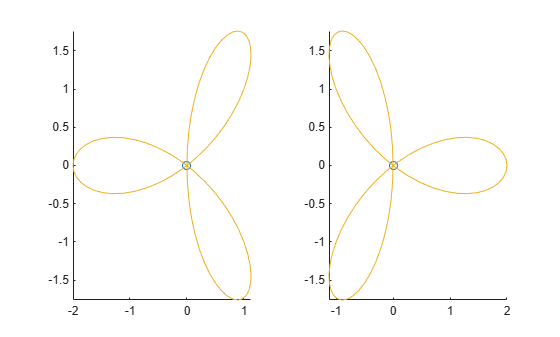# comet

2-D comet plot

## Syntax

``comet(y)``
``comet(x,y)``
``comet(x,y,p)``
``comet(ax,x,y,p)``

## Description

````comet(y)` displays a comet plot of the data specified in `y` versus the index of each value. A comet is an animation of a marker (head) and a line (tail) tracing a growing line over the data points. The tail is a solid line that traces the entire function.```

example

````comet(x,y)` displays a comet plot of `y` versus `x`.```

example

````comet(x,y,p)` specifies the comet body length. The comet body is a trailing segment in a different color that follows the head before fading. The body length is `p*length(y)`, where p is a scalar in [0, 1). ```

example

````comet(ax,x,y,p)` plots the comet into the axes specified by `ax`.```

## Examples

collapse all

Create a comet plot of data in `y` versus data in `x`. Create `y` as a vector of sine function values for input values between 0 and $2\pi$. Create x as a vector of cosine function values for input values between 0 and $2\pi$. Use an increment of $\pi /100$ between the values. Then, plot the data.

```t = 0:pi/100:2*pi; y = sin(t); x = cos(t); comet(x,y)```Create a comet plot and specify the comet body length by setting the scale factor input `p`. The comet body is a trailing segment in a different color that follows the head before fading.

Create `x` and `y` as vectors of trigonometric functions with input values from 0 to $4\pi$. Specify `p` as 0.5 so that the comet body length is `0.5*length(y)`. Then, plot the data.

```t = 0:pi/50:4*pi; x = -sin(t) - sin(t/2); y = -cos(t) + cos(t/2); p = 0.5; comet(x,y,p)```Create two comet plots in a tiled chart layout by specifying the target axes for each plot. Create two data sets, `x1` and `y1` and `x2` and `y2` as vectors of trigonometric functions with input values from 0 to $4\pi$. Specify the body length scale factor `p` as 0.25 so that the body length is `0.25*length(y)`.

```t = 0:pi/20:4*pi; x1 = -cos(t) + cos(t/2); y1 = -sin(t) - sin(t/2); x2 = cos(t) - cos(t/2); y2 = -sin(t) - sin(t/2); p = 0.25;```

Store the two `Axes` objects as `ax1` and `ax2`. Specify the target axes for each comet plot by including the `Axes` object as the first input argument to `comet`.

```tiledlayout(1,2); ax1 = nexttile; ax2 = nexttile; comet(ax1,x1,y1,p) comet(ax2,x2,y2,p)```## Input Arguments

collapse all

y-values, specified as a scalar or vector. To plot against specific x-values you must also specify `x`. If you specify geographic axes for `ax`, then `y` represents longitude in degrees.

x-values, specified as a vector. `x` and `y` must be the same length. If you specify geographic axes for `ax`, then `x` represents latitude in degrees.

Comet body length scale factor, specified as a value in the interval [0, 1). The comet body length is `p*length(y)`.

Target axes, specified as an `Axes` object or `GeographicAxes` object.

## Limitations

• Comet plots do not support data tips.